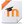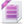Topic Name DescriptionDownload a copy of this courseDownload the backup imscc for this course. (For LMS other than Moodle)Course Feedback/Report an IssueCourse AttributionPhysics Year At A Glance
Introduction to PhysicsUnit 1 Planning Guide

This is the teacher's guide for the first unit.Making and Using MeasurementsProblem Solving In Physics

This presentation will teach you 5 steps to use to solve any problems in physics.

Newton's First LawUnit Plan

Teacher's Guide to the Newton's First Law Unit.First Law Introduction

Use this presentation to learn about the behavior of objects that have balanced forces.Speed and Velocity Introduction

Learn how to do problems involving constant velocity.

Newton's Second LawUnit Plan

Teacher's planning guide for the Newton's Second Law UnitNewton's Second Law Introduction

Learn about an object's motion when it has unbalanced forces.Accelerated Motion Introduction

Learn about the equations that describe the motion of objects undergoing constant acceleration.

Two Dimensional Motion UnitUnit Plan

Teacher planning guides for the Two Dimensional Motion Unit.Vector Introduction

Learn how quantities add when they have both magnitude and direction.Projectile Introduction

Learn to describe and predict the motion of objects hurtling through the air!Circular Motion Introduction

Lean about the physics that describes objects that are moving in a circular path.

Newton's Third LawUnit Plan

Teacher's planning guide for the Newton's Third Law UnitThird Law Intro

Learn about the relationship of action/reaction pairs of forces.Momentum Introduction

Learn about momentum, a way to predict the results of collisions between objects.

EnergyUnit Plan

Teacher's planning guide for the Energy Unit.Energy Introduction

Learn about the formulas that relate the different forms of energy.

Mechanical WavesUnit Plan

Teacher's Planning guide for the Mechanical Waves UnitMechanical Wave Introduction

Learn about the characteristics of waves and their interactions.

Electromagnetic WavesUnit Plan

Teacher's planning guide for the Electromagnetic waves unit.Electromagnetic Wave Introduction

Electricity and MagnetismUnit Plan

Teacher's planning guide for the Electricity and Magnetism unit.Circuit Introduction

Learn about the components of an electric circuit.

Modern Physics ProjectsUnit Plan

Teacher's Planning Guide for the Modern Physics Activities.Particle Physics Introduction

Use this presentation to learn a little bit about particle physics.